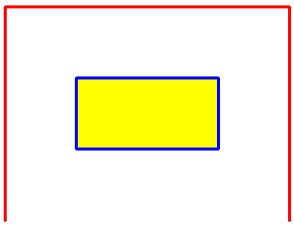# QGIS Python Tutorial: Drawing Polyline and Polygon

In another post has been discussed how to draw marker in QGIS map canvas. Maybe you have a question what about polyline and polygon? How to draw those in QGIS using Python? This tutorial will give the answer.

### Drawing Polyline

To draw a polyline we are using QgsRubberBand class. But Firstly we need to initiate the canvas first using QGIS interface.

```canvas=iface.mapCanvas()
```

Using QgsPoints class then a list of points coordinate for a polyline are defined. Then we set the geometry with QgsGeometry class and set the defined points as the argument. Finally customize  how the polyline looks like. Set it's color and the width of the polyline. The complete code as follow:

```#Drawing Polyline
polyline = QgsRubberBand(canvas, False)  # False = not a polygon
points =[QgsPoint(-124,48), QgsPoint(-124,51 ), QgsPoint(-120,51),QgsPoint(-120,48)]
polyline.setToGeometry(QgsGeometry.fromPolyline(points), None)
polyline.setColor(QColor(255, 0, 0))
polyline.setWidth(3)
```

### Drawing Polygon

Similar with polyline, we also use QgsRubberBand class to draw polygon. But in defining the point list we are using QgsPointXY class instead of QgsPoint. Then we customize the outline color of the polygon, it's widht and fill color. The complete code to draw polygon can be seen below.

```#Drawing Polygon
polygon = QgsRubberBand(canvas)
p_points=[QgsPointXY(-123, 49), QgsPointXY(-123, 50),QgsPointXY(-121, 50),QgsPointXY(-121,49)]
polygon.setToGeometry(QgsGeometry.fromPolygonXY([p_points]),None)
polygon.setColor(QColor(0, 0, 255))
polygon.setFillColor(QColor(255,255,0))
polygon.setWidth(3)
```

Figure 1 shows the polyline and polygon from the code above. We can see a red polyline and a yellow polygon with blue outline in QGIS map canvas. To show and hide the drawing object use hide and show method. Type it in the console.

```polyline.hide() # to hide polygon
Polyline.show() # to show polygon
```Figure 1. Polyline and Polygon drawing
That's all the tutorial how to add polyline and polygon with python in QGIS. Please see other tutorials which discuss other topics in the QGIS python programming tutorial series. To learn further about this topic, take a look the PygGIS Developer Cookbook.